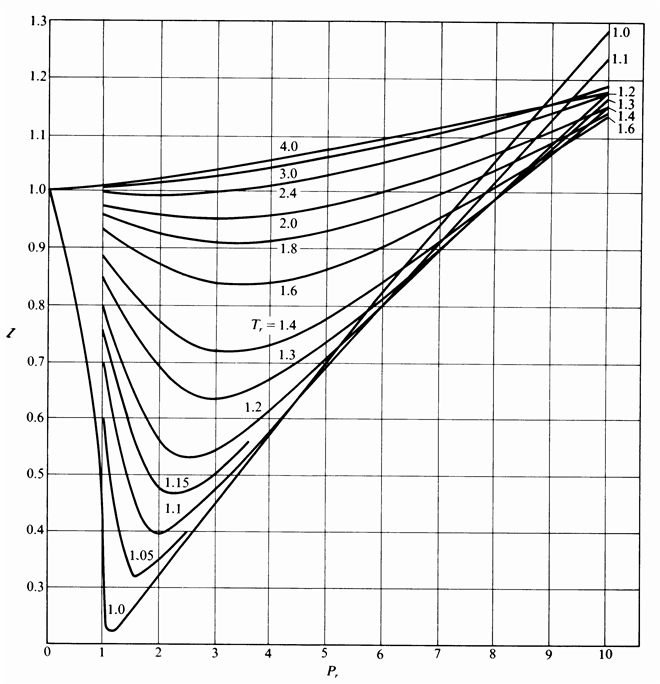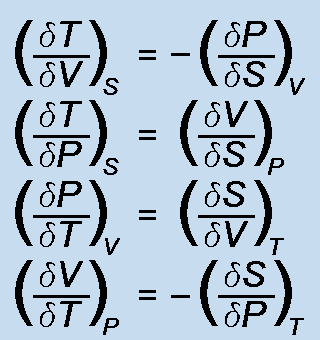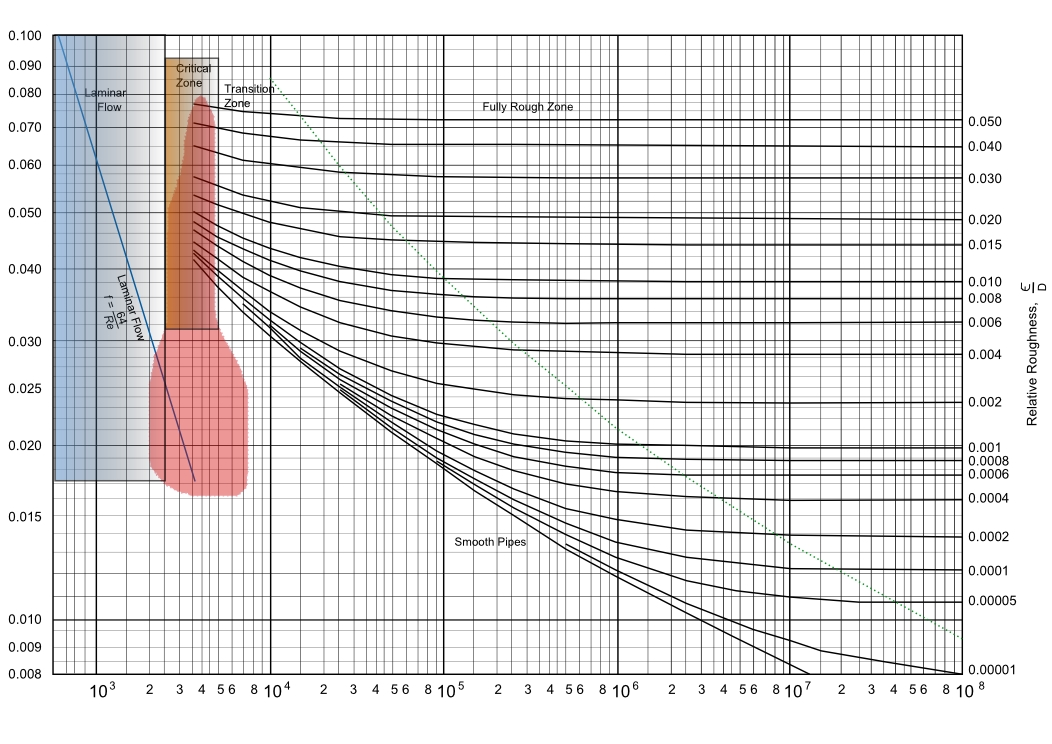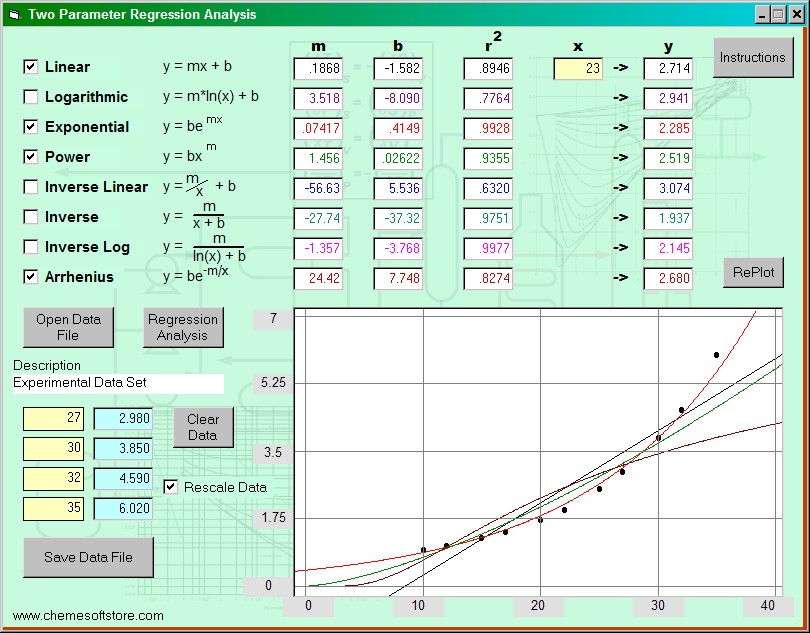### ChemE Soft StoreCaCO3 -> CaO + CO2
NaCl + NH3 + CO2 + H2O -> NaHCO3 + NH4Cl
2 NaHCO3 -> Na2 CO3 + H2O + CO2
CaO + H2 O -> Ca(OH)2
Ca(OH)2 + 2 NH4 Cl -> CaCl2 + 2 NH3 + 2 H2O

## 2 Parameter Regression

Two parameter regression analysis solving for eight different functions. Functions are plotted as well as correlation coefficients calculated. Functions include the temperature dependent Arrhenius equation, and inverse functions.#### Features:

• Data sets can be saved as separate text files, editable by notepad programs
• Correlation coefficients calculated for all functions shown for easy comparison
• Any function can be plotted for visual comparison
• Y values of all functions can be calculated for any input x value
• Instructions for the software application is presented